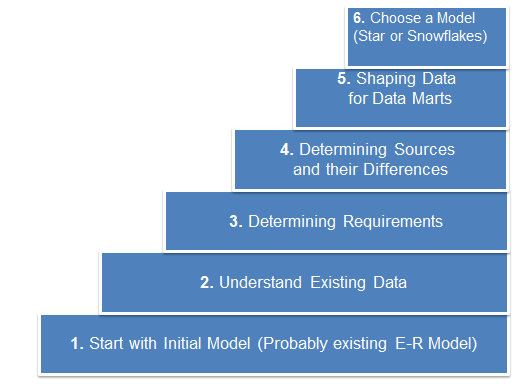## Monday, October 1, 2012

### Part 4.3 Dimensional Data Model

4.3 Dimensional Data Modeling

4.3.1      Steps in Dimensional Modeling
4.3.2      Star Schema & Snowflake Schema
4.3.3      The Implementation Approach (Bill & Ralph)
4.3.4      Dimensional Modeling Fundamentals
4.3.4.1   Dimensional Modeling Overview
4.3.4.2   Dimensional Modeling Steps
4.3.5     Confirmed Dimensions
4.3.6     Slowly Changing Dimension
4.3.8     Dimensional Modeling framework

4.3.1 Steps in Dimensional Modeling4.3.2 Star Schema & Snowflake Schema4.3.3 The Implementation Approach (Bill & Ralph)

4.3.4 Dimensional Modeling Fundamentals

4.3.4.1 Dimensional Modeling Overview

Dimensional Modeling

• A logical design technique that seeks to present the data in a standard framework that is intuitive and allows for high performance access
• It is inherently dimensional and adheres to a discipline that uses relational model with some important restrictions
• The fundamental idea of dimensional modeling is that nearly every type of business data can be represented as a kind of cube of data, where the cells of the cube contain measured values and the edges of the cube define the natural dimensions of the data
• Every dimensional model is composed of one table with multipart key, called the fact table, and a set of smaller tables called dimension tables
• Each dimension table has a single part primary key that corresponds exactly to one of the components of the multipart key in the fact table
• This characteristic star-like structure is often called a star join
• The fact table contains facts or measurements of the business
• The dimension tables contain textual attributes that describe the facts
• The attributes in the dimension tables are used for constraining & grouping data within data warehouse queries

Dimension Tables

• Dimension Tables are the entry points into the data warehouse
• Dimension tables are designed especially for selection and grouping under a common head
• Determine contextual background for facts
• Parameters for OLAP
• Common Dimensions are D
Date

Product

Location/Region

Customers
Fact Table

The fact table is where the numerical measurements (measure)of the business are stored

Facts

• The detail information in a Fact tables,For Examples: Sales Quantity, Unit Sales Price, Sales Amount etc.
• Key performance indicators of the business
• Numeric in Nature
• Analyzed across the dimensions

4.3.4.2 Dimensional Modeling Steps

Dimensional Modeling Steps

1. To build a dimensional database Choose the business processes that you want to use to analyze the subject area to be modeled.
2. Determine the Granularity of the fact tables.
3. Identify Dimensions and Hierarchies for each fact table.
4. Identify Measures for the fact tables.
5. Determine the Attributes for each dimension table.
6. Get users to verify the data model.

4.3.5 Confirmed Dimensions

4.3.6 Slowly Changing Dimension

Semi Additive - Not additive across one or more dimensions (usually across time). Need to query using single unit of non-additive dimension.

Non-Additive - Non-additive. Store individual components and calculate ratio of sums vs. sum of ratio’s.

4.3.8 Dimensional Modeling framework

﻿

#### 1 comment:

1.